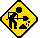## Sharp EL-5500

Datasheet legend
Ab/c: Fractions calculation
AC: Alternating current
BaseN: Number base calculations
Card: Magnetic card storage
Cmem: Continuous memory
Cond: Conditional execution
Const: Scientific constants
Cplx: Complex number arithmetic
DC: Direct current
Eqlib: Equation library
Exp: Exponential/logarithmic functions
Fin: Financial functions
Grph: Graphing capability
Hyp: Hyperbolic functions
Intg: Numerical integration
Jump: Unconditional jump (GOTO)
Lbl: Program labels
LCD: Liquid Crystal Display
LED: Light-Emitting Diode
Li-ion: Lithium-ion rechargeable battery
Lreg: Linear regression (2-variable statistics)
mA: Milliamperes of current
Mtrx: Matrix support
NiMH: Nickel-metal-hydrite rechargeable battery
Prnt: Printer
RTC: Real-time clock
Sdev: Standard deviation (1-variable statistics)
Solv: Equation solver
Subr: Subroutine call capability
Symb: Symbolic computing
Tape: Magnetic tape storage
Trig: Trigonometric functions
Units: Unit conversions
VAC: Volts AC
VDC: Volts DC
 Years of production: Display type: Alphanumeric display New price: Display color: Black Display technology: Liquid crystal display Size: 3"×7½"×½" Display size: 24 characters Weight: 5 oz Entry method: BASIC expressions Batteries: 2×"CR-2032" Lithium Advanced functions: Trig Exp Hyp Lreg Cmem Snd External power: Memory functions: + I/O: Interface port Programming model: BASIC Precision: 12 digits Program functions: Jump Cond Subr Lbl Ind Memories: 6(0) kilobytes Program display: Text display Program memory: 6 kilobytes Program editing: Text editor Chipset: Forensic result:The Sharp EL-5500 is a vintage BASIC calculator.As usual, I wrote a Gamma function program to test the capabilities of this machine. In it, I made use of the DATA statement to simplify the program a little. Here's the result:

```10:DATA 76.18009172,9.5E-9
20:DATA -86.50532032,-9.4E-9
30:DATA 24.01409824,8E-10
40:DATA -1.231739572,-4.5E-10
50:DATA 1.208650973E-3,8.7E-13
60:DATA -5.395239384E-6,-9.5E-16
100:INPUT "X=",X
110:T=1
120:IF X>=0 THEN 160
130:T=T*X
140:X=X+1
150: GOTO 120
160:G=1.9E-10+1
170:FOR I=1 TO 6